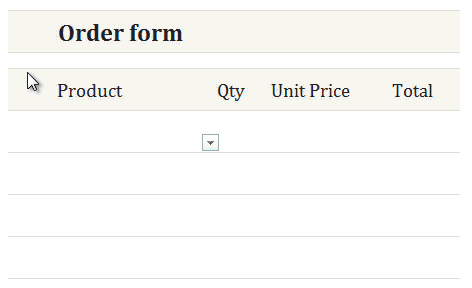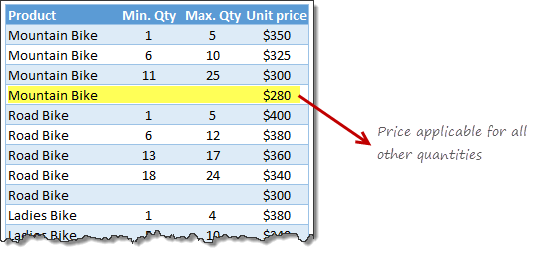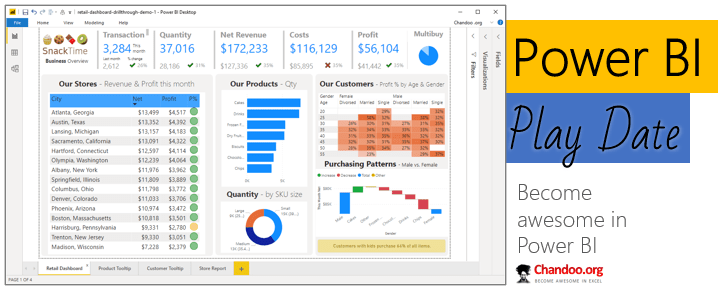# Pricing Tier Lookup formula

Share

Here is an interesting twist on the good old VLOOKUP. How to find the pricing applicable for given quantity of a product?

Something like this:### Writing pricing tier lookup formula:

Meet the data:

Let’s say you have data like this:Assume the data is in a table named pricing

PLOOKUP formula:

Okay, I am kidding. There is no PLOOKUP formula (P for Price). But we can whip our own version of PLOOKUP using other powerful formulas in Excel.

Let’s say we have product name in cell C6 and quantity in cell D6.

To find the applicable unit price of D6 units of C6 (for example 12 units of Mountain Bikes),

We can use below formula:

`=SUMIFS(pricing[Unit price], pricing[Product],C6, pricing[Min. Qty],"<="&D6, pricing[Max. Qty], ">="&D6)`

Remember: product prices are in the table named pricing.

How does this formula work?

This is a simple SUMIFS formula that looks at the unit price column of pricing table and sums up all unit prices that match with given product name (C6), with quantity (D6) between [Min. Qty] and [Max. Qty] columns.

In other words, this SUMIFS will narrow down to the one row containing Mountain Bike (11-25) tier and returns the price as \$300, if our input is 12 units of Mountain Bike.

Oh wait..! What if the quantity is 32?

You are right, our SUMIFS is too lame to handle cases where the input quantity doesn’t fit in any of the tiers.

But, we can use a simple logic to fix this problem.

Here is the final formula that works in all situations:

`=MAX( SUMIFS(pricing[Unit price], pricing[Product],C6, pricing[Min. Qty],"<="&D6, pricing[Max. Qty], ">="&D6),SUMIFS(pricing[Unit price],pricing[Product],C6,pricing[Min. Qty],""))`

As you can see, we try to find the MAXIMUM of our original SUMIFS and a second SUMIFS that just looks at given product (C6) and blank value for [Min.Qty] column.

Of course, this assumes that prices go down with each tier. If your case is different, you need to alter the formula.

Click here to download the example workbook. Examine the formulas and play with input data to learn how this works. There are two bonus goodies in the workbook.

### More variations of lookup problems:

Here are few more ways to lookup tricky data:

### How would you write PLOOKUP?

While the SUMIFS approach works well, it does feel a bit long. Can you think of other ways to write pricing tier lookup formula? Post your answers in the comments section. Teach us something new.

### Get FREE Excel + Power BI Tips

Simple, fun and useful emails, once per week.

Learn & be awesome.

### Welcome to Chandoo.org

Thank you so much for visiting. My aim is to make you awesome in Excel & Power BI. I do this by sharing videos, tips, examples and downloads on this website. There are more than 1,000 pages with all things Excel, Power BI, Dashboards & VBA here. Go ahead and spend few minutes to be AWESOME.

Read my storyFREE Excel tips book

Chandoo is an awesome teacher
5/5

– Jason

Still on fence about Power BI? In this getting started guide, learn what is Power BI, how to get it and how to create your first report from scratch.### Introducing Power BI Play Date – Online class to master Power BI (oh yeah, Power Query & Power Pivot too)

Hello friends,

Check out below slideshow to see some of the reports you can create in our course. It is #AWESOME.

## Related Tips

### 14 Responses to “Pricing Tier Lookup formula”

1.AMIT SHARMA says:

nice you always bring new trick for us in excel and we learn you tricks thanks yaar

2.jason m says:

I think that this is a situation where I would fill in the gaps in the table to simplify the formula. Rather than blanks for "Mountain Bike" when the Qty. is > than 25, I would put 26 for "Min." and 99999 for "Max." and so on for the other bikes. That would simplify the SUMIFS or SUMPRODUCT formula:

=SUMPRODUCT((G12=pricing[Product])*(H12>=pricing[Min. Qty])*(H12<=pricing[Max. Qty])*(pricing[Unit price]))

3.Nad says:

After filling the gaps in Min. Qty column adding 1 to the previous Max. Qty (there's no need to fill in the gaps in Max Qty column), use Vlookup to get the price (array formula):

=VLOOKUP(H11,IF(pricing[Product]=G11,pricing[[Min. Qty]:[Unit price]],""),3)

4.Nad says:

I removed first row in Chandoo's sheet. The right formula is:

=VLOOKUP(H12,IF(pricing[Product]=G12,pricing[[Min. Qty]:[Unit price]],""),3)

5.Mike Hansford says:

SLightly longwinded.... I also had to add a a break into the top price band so eg. C8 on the data tab is 26, cell C13 is 25 and so forth

=IF(C9="",0,VLOOKUP(D9,INDIRECT("data!C"&MATCH(C9,data!B:B,0)&":E"&(COUNTIF(data!B:B,plookup!C9))-1+MATCH(C9,data!B:B,0)),3,TRUE))

•Mike Hansford says:

I missed the alternative formukla cell. My formula was in cell C9 in the Plookup sheet

6.David N says:

For those who prefer INDEX-MATCH, this formula for finding Unit Price can also ignore Max. Qty altogether once the final Min. Qty has been entered...

{=INDEX(pricing[Unit price],MATCH(D6,(pricing[Min. Qty])/(pricing[Product]=C6),1))}

...and an extra INDEX can make it non-array...

=INDEX(pricing[Unit price],MATCH(D6,INDEX((pricing[Min. Qty])/(pricing[Product]=C6),0),1))

7.Haz says:

Assuming that the price goes down every tier, you don't even need the Min. Qty

={MAX((pricing[Product]=C6)*(pricing[Max. Qty]+(pricing[Max. Qty]=0)*D6>=D6)*pricing[UnitPrice])*D6}

8.Dave Patton says:

Consider array formula
=MIN(IF(B5:B23=G14,IF(C5:C23<=H14,E5:E23)))

or the relatively new function

=AGGREGATE(15,6,E5:E23/((B5:B23=G15)*(C5:C23<=H15)),1)

•Ferdinand Santos says:

First of all, thanks for the formulas. I really like the AGGREGATE function. Never used it before.

Is this doable using Power Query?

9. […] Pricing Tier Lookup Using Sumifs instead of lookups  post by Chandoo […]

10.SteveJ says:

I need some rookie help here. I'm trying to replicate this in an existing spreadsheet with a different named range (Price) and headers [Price, Begin Date, End Date]. But when I replace the existing formula with my range and header names, I get an error at the first range/name combination in the formula. On the existing that would be at: pricing[Unit price] after =Max(SUMIFS(.
Can someone tell me why I can't get this to work? I tried a few of the other formulas in this string as well and I can get some to work in the example spreadsheet, but not in my spreadsheet. Thanks,

•SteveJ says:

i.e., I'M the Rookie and I need some Expert help please. thx!

•SteveJ says:

Nevermind. Just realized that the data is formatted as a named Table, not just a named range.

### Get FREE Excel & Power-BI Newsletter

One email per week with Excel and Power BI goodness. Join 100,000+ others and get it free.